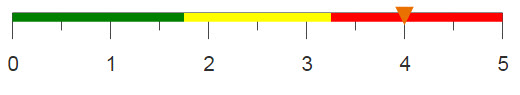# Combining rows of multiple tables

You can efficiently combine the rows of multiple tables that are located in the same folder and have the same columns using <loop>. The result will be a single worksheet containing the rows from each table.

## Difficulty## Objective

You have a folder containing multiple tables that have the same columns but which have data from different years. You would like to combine the rows of each table into a master table, and you would like each row to indicate which year it is from. While it is possible to simply perform a <merge> operation for each table you'd like to include, new tables added to the folder in the future will not be accounted for, and your code could become lengthy. Instead, utilizing the <loop> operation allows you to perform the same <merge> operation on every table in the folder. This method will also allow you to include any tables that might be added in the future without having to modify the code.

## Solution

```<loop with_="files">
<outer>
<directory folder="pub.demo.weather"/>
<colord cols="path"/>
<col name="path" format="width:50"/>
<sel value="contains(path;'pub.demo.weather.hourly')"/>
<transpose/>
</outer>
<inner>
<base table="{@files.m0}"/>
<letseq tables="{@files}" keys="{pkg_names(@tables)}">
<tabu label="Tabulation on Hourly U.S. Weather" breaks="id,date">
<tcol source="prec" fun="avg"
label="Average`Hourly`Precip`(.01 inch)"/>
<tcol source="snow" fun="avg"
label="Average`Snow`Depth`(inches)"/>
<tcol source="temp" fun="avg"
label="Average`Dry Bulb`Temp`(Celsius)"/>
</tabu>
<foreach table_year="{@keys}" tally_="@i">
<if test="{@i>1}">
<then>
<merge table2="{@files.{@table_year}}" match="pad">
<tabu label="Tabulation on Hourly U.S. Weather"
breaks="id,date">
<tcol source="prec" fun="avg"
label="Average`Hourly`Precip`(.01 inch)"/>
<tcol source="snow" fun="avg"
label="Average`Snow`Depth`(inches)"/>
<tcol source="temp" fun="avg"
label="Average`Dry Bulb`Temp`(Celsius)"/>
</tabu>
</merge>
</then>
<else/>
</if>
</foreach>
</letseq>
</inner>
</loop>```

## Alternate solution

```<loop with_="files">
<outer>
<directory folder="pub.demo.weather"/>
<colord cols="path"/>
<col name="path" format="width:50"/>
<sel value="contains(path;'pub.demo.weather.hourly')"/>
<transpose/>
</outer>
<inner>
<base table="default.lonely"/>
<letseq tables="{@files}" keys="{pkg_names(@tables)}">
<foreach table_year="{@keys}" tally_="@i">
<merge table2="{@files.{@table_year}}" match="pad">
<tabu label="Tabulation on Hourly U.S. Weather" breaks="id,date">
<tcol source="prec" fun="avg" label="Average`Hourly`Precip`
(.01 inch)"/>
<tcol source="snow" fun="avg" label="Average`Snow`Depth`
(inches)"/>
<tcol source="temp" fun="avg" label="Average`Dry Bulb`Temp`
(Celsius)"/>
</tabu>
</merge>
</foreach>
</letseq>
<sel value="date<>NA"/>
<colord hide="c1"/>
</inner>
</loop>```

## Discussion

The <loop> operation is a useful way to perform the same actions repeatedly without the need to duplicate code. This recipe uses <loop> in conjunction with the <merge> operation to merge several tables in the same folder. In this way, you can combine numerous tables without needing to use multiple <merge> operations.

Both of the above solutions use the <directory> operation to first create a table containing the information for all of the tables within a specific folder. Once that information is obtained, the <colord> operation is used to reduce the worksheet's contents to a single column of table names. Since the folder contains additional tables that should not be included in the merged table, the <sel> operation is used to select only the rows that contain the names of the desired tables. Then, the <transpose> operation converts the worksheet from a single column to a single row, and the values are placed in a package by the <outer> part of the <loop> in the variable specified by the with_ attribute.

In the main solution, the <inner> loop then specifies a base table using the first item in the package ({@files.m0}), and the <foreach> operation merges each additional item. In order to avoid merging the base table to itself, you can use an <if> statement to only merge after the first iteration. An initial tabulation is performed to aggregate the data into the desired format, and the same tabulation is then performed on each subsequent table before the final results are merged.

Alternatively, you can use an empty table, default.lonely, as the base table to which all other tables are merged. This will create an empty row at the top of the data table and an empty column labeled c1. However, these can be easily removed by using the <sel> and <colord> operations after the merge.

## Further reading

If you would like to learn more about the functions and operations discussed in this recipe, click on the links below:

<loop>

<merge>

<foreach>